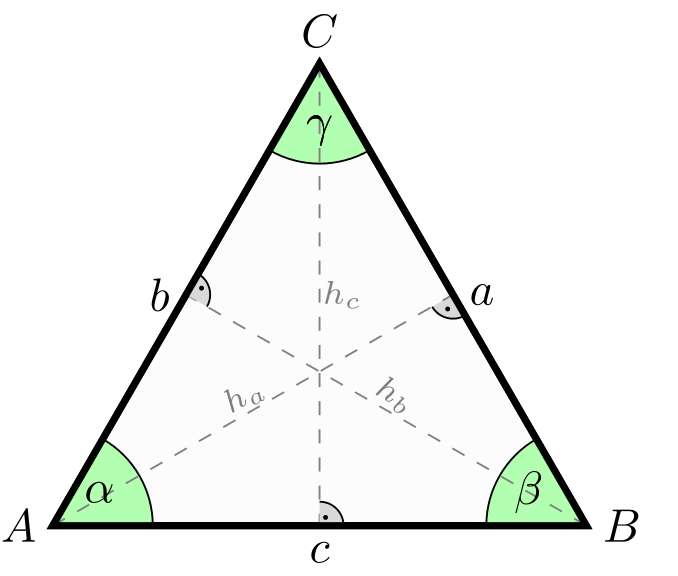# Triangle Calculator with two angles and one opposite side

Instructions: Use this triangle calculator to completely determine a triangle in the AAS case. Please provide the two angles and a side that is opposite to one of the angles (arrange the angles so that the angle that has the opposite side known is provided as the first angle):Angle 1 (choose degrees or radians) =Angle 2 (choose degrees or radians) =Side (Valid positive expression. Ex. 3, or 1/3, etc) =

## How to Solve a Triangle Knowing Two Angles and One Opposite Side

This triangle calculator will show you all the steps required to resolve a triangle (the sides and angles), when knowing only a portion of the information about the triangle. In this specific case, it is assumed that you know two angle and one side that is opposite to one the angles, and we assume without loss of generality that it is opposite to the first angle.

By now you know that strategy used to resolve the triangle will depend on what information you have available. In this case you have the AAS case(where as you know "S" stands for side and "A" for angle).### Is the AAS the easiest case to solve the triangle?

Perhaps, but it is not different from other cases where you have to use both the Law of Sines and Law of Cosines. So, it is not really clear which one is the easiest one, what is clear is that you need to be able to depict your specific situation graphically, and decide which is the right tool to use

It is either that you need to use the Law of Sines, the Law of cosines or the law that says that the sum of the three angles will be 180o degrees (or $$2\pi$$ radians).

### Other triangle resolution cases

For other cases, you can use the triangle solver for three sides of the triangle solver for SAS case, that will work for different situations.